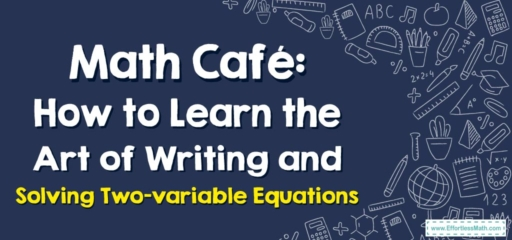# Math Café: How to Learn the Art of Writing and Solving Two-variable Equations

Welcome back to the Math Café, friend!

Today's special is a heart-warming topic that many find filling and satisfying - Writing and Solving Two-variable Equations. So, pull up a chair, grab a cup of coffee, and let's enjoy the delicious math that lies ahead.## 1. Our Today’s Special: Two-variable Equations

Two-variable equations are our math dish for the day. They’re equations that involve two different variables (like ‘$$x$$’ and ‘$$y$$’). An example of a two-variable equation is $$y = 2x + 3$$.

## 2. The Recipe: Writing and Solving

Our recipe involves two main steps: writing the equation and then solving it. It’s like baking a cake: first, we mix our ingredients (write the equation), and then we bake it to perfection (solve the equation).

### Your Personal Chef Guide: How to Write and Solve Two-variable Equations

Let’s get cooking:

#### Step 1: Writing the Equation

This is where we translate a word problem or a real-life scenario into a two-variable equation. Look for the relationships and patterns that link the variables together.

#### Step 2: Solving the Equation

Now, we use algebraic methods to solve the equation for one of the variables. The solution will be in terms of the other variable.

Consider this problem: “If twice a number, increased by $$3$$, equals that number plus $$10$$. What is the number?”

1. Writing the Equation: “Twice a number” suggests $$2x$$, “increased by $$3$$” suggests $$+\ 3$$, “equals to that number plus $$10$$” gives us $$= x + 10$$. This translates into the equation $$2x + 3 = x + 10$$.
2. Solving the Equation: Subtract x from both sides to get $$x + 3 = 10$$. Then, subtract $$3$$ from both sides to solve for $$x$$, giving us $$x = 7$$.

That’s it for today’s special at the Math Café! Writing and solving two-variable equations can be a piece of cake with enough practice. So, keep working on your math recipes and before you know it, you’ll be a math gourmet. Stay curious, keep learning, and see you at our next math café gathering!

### What people say about "Math Café: How to Learn the Art of Writing and Solving Two-variable Equations - Effortless Math: We Help Students Learn to LOVE Mathematics"?

No one replied yet.

X
30% OFF

Limited time only!

Save Over 30%

SAVE $5 It was$16.99 now it is \$11.99